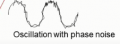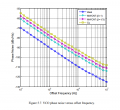# problem imagening the meaning of phase noise definition -70dbc/hz at 10Khz

#### yef smith

Joined Aug 2, 2020
568
Hello,From Wikipedia i have the following definition, but i cant imgine it.
Noise is not deterministic it has a variance and other properties of a random type.

dBc means ratio in amplitude between the carrier and some point
here we have some random thing so how ding /HZ helps us catch soe value from it?
https://en.wikipedia.org/wiki/Phase_noise

Phase noise (ℒ(f)) is typically expressed in units of dBc/Hz, and it represents the noise power relative to the carrier contained in a 1 Hz bandwidth centered at a certain offsets from the carrier. For example, a certain signal may have a phase noise of −80 dBc/Hz at an offset of 10 kHz and −95 dBc/Hz at an offset of 100 kHz. Phase noise can be measured and expressed as single-sideband or double-sideband values, but as noted earlier, the IEEE has adopted the definition as one-half of the double-sideband PSD.

#### Papabravo

Joined Feb 24, 2006
20,386
Well put it another way. The noise power in watts is 7 orders of magnitude smaller than the carrier. So if we have 1 watt of carrier power we have 100 nanowatts of noise power because:

$$10\;\cdot\;log \left(\cfrac{100\; \times\;10^{-9}}{1}\right)\;=\;-70\;\text {dBc}$$

Does that help?
The nice thing about using power is the process of squaring and integrating reduces the randomness to a measurable quantity that you can relate to.

#### Papabravo

Joined Feb 24, 2006
20,386
Power density, or more properly "Power Spectral Density" is still power, but as a function of frequency. It can be expressed in watts, or rendered in dimensionless relative units like dB

#### sparky 1

Joined Nov 3, 2018
735In the frequency domain we might see center frequency and side lobes as an ideal without artifacts
A typical distortion in the time domain an oscilloscope set to the carrier frequency shown above
A semiconductor chip may have remedied some of the phase noise, very close but not ideal shown below.
Data taken from spec analyzer ported into graph shows chip topology advancement in reducing phase noise.Last edited: